# What Are Odds? The Right Way To Look At Odds For Sports Betting

### UNDERSTANDING ODDS AND PROBABILITY

In most places related to sports and betting you will see the odds of a team winning rather than the probability.

The reason you will see odds instead of probability, is because the odds tells you how much you win if you placed a bet on the given odds.

E.g. if you bet $100 @ 2.00 in odds, you would get$200 in return if you won.

One could have used probability instead to describe how likely it was that you would win the bet.

Andrew has now bet $100 on the odds of 1.25, giving him a potential return of$125, or a profit of $25.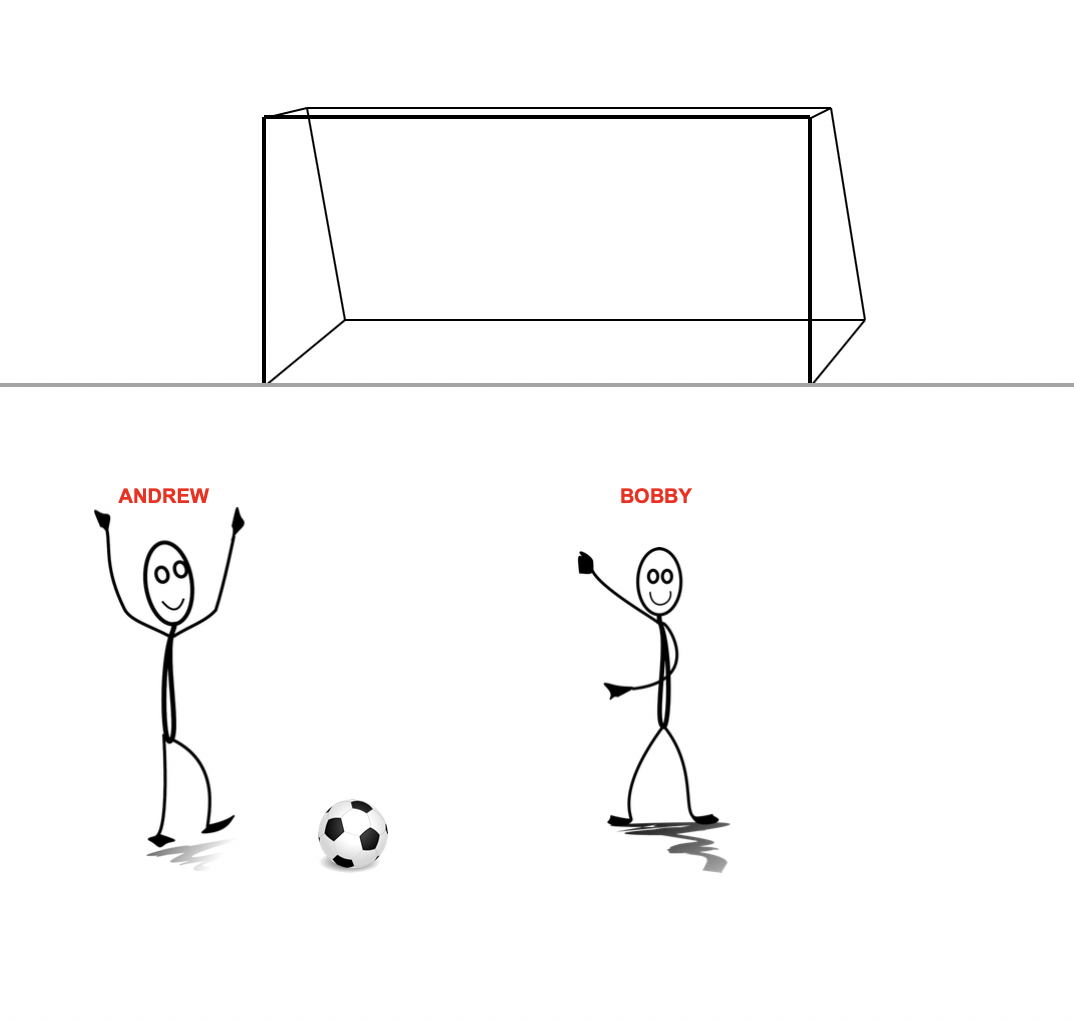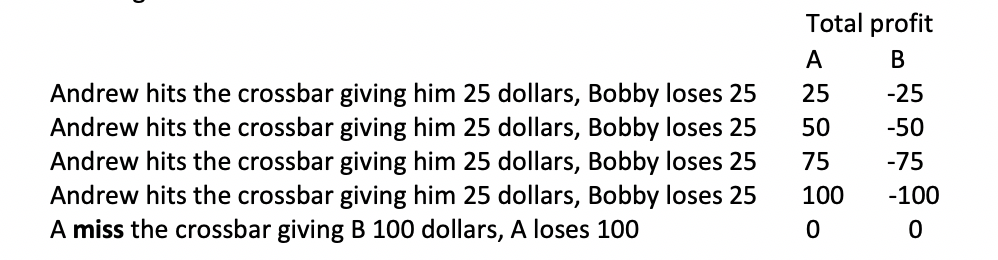In a sample size of only 5 kicks to hit the crossbar, this would generally not happen because the variance (or standard deviation) is too high. But, when you do this over a large sample size, say he is kicking the ball 100,000 times, the variance becomes smaller and the probability that is reflected in the odds correlates much better with the probability for the real events. If this is confusing you should read more about the law of large numbers. It is important to notice that they would break even only for events where the odds and probability relates to each other e.g. a coin flip. ### A BET WITH NO EXPECTED VALUE (-EV) Bobby now gets a downgrade in IQ, so he does not understand the math.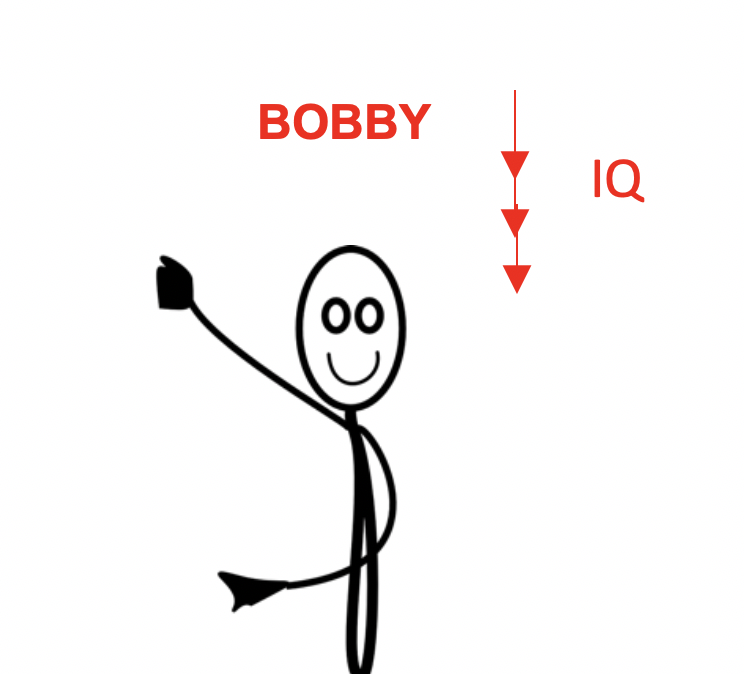Andrew then asks again, what odds Bobby can provide if he wants to bet$100. Bobby says he would give him 1.5 for hitting the crossbar, while giving himself the odds of 3.:

But, if the odds were to relate to the probability, we would give the odds of 1.25 and 5, as in the last example. Let’s see what happens with 1.5 and 3 in odds:

Andrew still bets $100, with a potential return of$150, or a profit of $50. Bobby is betting$50, with a potential return of $150, or a profit of$100.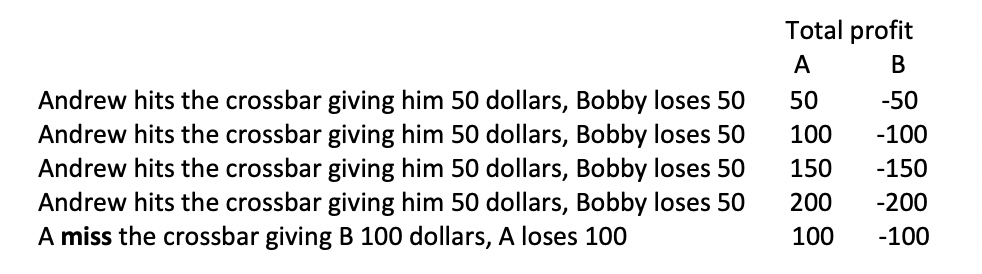As we can see, A wins, while B loses in the long term. This is because the odds of him hitting the bar is overvalued (or the underlying probability is undervalued) while the odds of him missing is undervalued (or the underlying probability is overvalued).

When the probability is undervalued it means that the event happen more often than the probability suggests in the odds.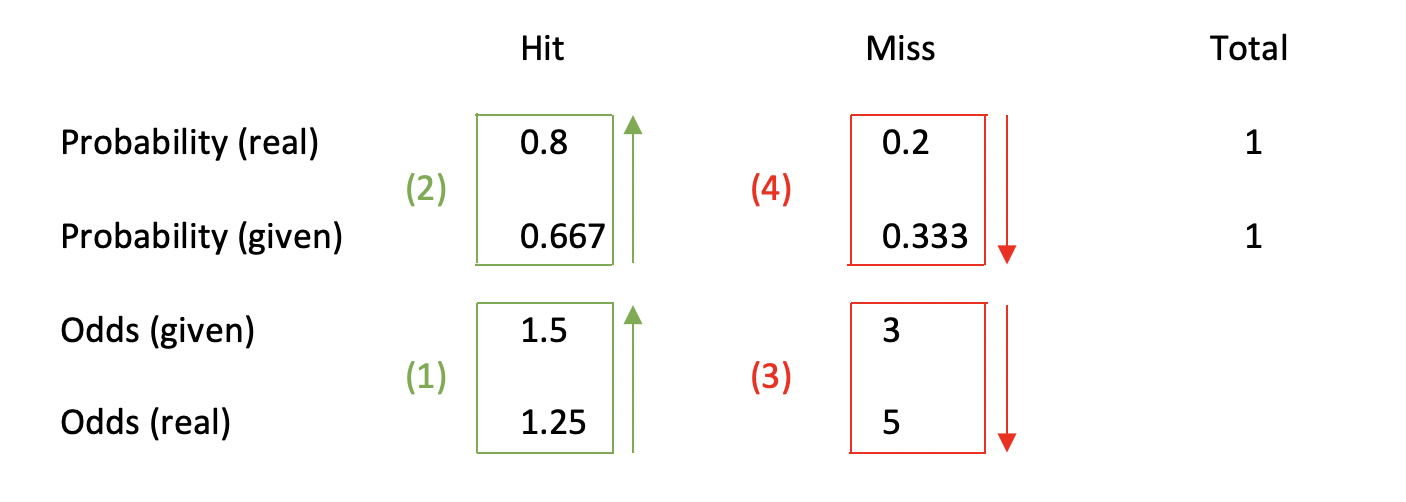But then you might want to ask: But no sports match is the same, so how is this applicable to the real world?

Well, over a vast amount of games there is large evidence for the probability to be reflected in the odds. If you want to read more about it, check this article out.

### KEY TAKEAWAY

Odds and probability are in theory really just two sides of the same coin, but in practice the case is different.

This is because (soft) bookmakers usually offer unfair odds, which do not reflect the underlying probability.

This is the way the bookmakers make money, so it is important to understand that the odds at the bookmakers almost never reflect the underlying probability.

### DO YOU KNOW THE DIFFERENT ODDS TYPES?

We have written articles explaining them: